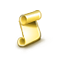Interesting script?
So post a link to it -
let others appraise it

You liked the script? Try it in the MetaTrader 5 terminal# Burg Extrapolator - expert for MetaTrader 5

Views:
1813
Rating:
Published:
2017.11.03 12:30
Updated:
2018.02.28 10:22

Author of the idea: Vladimir, mq5 code author: barabashkakvn.

The Expert Advisor uses Burg's method for linear prediction. Linear prediction is based on finding future values as linear functions of previous values. Suppose we have the x..x[n-1] price range where the higher index corresponds more recent prices. The prediction of the x[n] future price is calculated as follows:

x[n] = -Sum(a[i]*x[n-i], i=1..p)

here a[i=1..p] are model ratios, and p is the model order. Burg's method finds the a[] ratios by decreasing a mean-square error on the last training n-p bars.

### Input Parameters

• MaxRisk - maximum risk of all simultaneously performed deals.
• ntmax - maximum number of deals in one direction.
• MinProfit - minimal predicted profit at which positions will be opened.
• MaxLoss - maximal predicted loss at which positions will be closed.
• TakeProfit - Take Profit value.
• StopLoss - Stop Loss value.
• TrailingStop - the Trailing Stop function.
• PastBars - the number of previous bars used to predict future values.
• ModelOrder - the order of Burg's model as a fraction of the number of past bars (0..1).
• UseMOM - enables detrend of input data: mom(i)=log[p(i)/p(i-1)].
• UseROC - enables detrend of input data: roc=100*(p(i)/p(i-1)-1).

Only one of UseMOM and UseROC variables can be true, i.e. UseMOM=true AND UseROC=true is not allowed.

Like most of optimized Expert Advisors, Burg Extrapolator only works well on training bars. The Expert Advisor will steadily lose money without a constant re-optimization.

Translated from Russian by MetaQuotes Software Corp.
Original code: https://www.mql5.com/ru/code/19046Pending orders DOWN

The scripts places multiple pending orders lower than the current price.Pending orders UP

The scripts places multiple pending orders higher than the current price.Carbophos

The Expert Advisor sets a grid of pending Sell Limit and Buy Limit orders.TRIX ARROWS

The Triple Exponential Average (TRIX) indicator + the signal line + arrows at the intersection of the signal and the main lines.